Request a call back

# CBSE Class 10 Mathematics Previous Year Question Paper Term 1 2021 JSK-1-Set-4

Does the sound of Class 10 Maths intrigue you? Or, does it scare you away? All of these happen because it's not easy to handle the Board pressure for CBSE Class 10. But, we will surely help you to tackle the syllabus for Class 10 Maths by providing you with the Class 10 Revision NotesClass 10 Textbook SolutionsClass 10 Tests for all of the chapters available in CBSE Class 10 Maths. We know that scoring more marks in CBSE Class 10 Maths has never been this easy before. But, by referring to all of the study materials we provide at TopperLearning, you can easily score more marks in the Class 10 board examination.

The study materials are created by our subject experts that we offer for CBSE Class 10, very well know the syllabus and essential facts of CBSE Class 10. These study materials will help you understand all the CBSE Class 10 Maths concepts as we focus on providing solutions that simplify a subject's complex fundamentals. At TopperLearning, we believe in delivering quality solutions at a low cost, and we strictly follow the latest CBSE Class 10 syllabus. We make sure that these study materials are revised from time to time. Our TopperLearning packages involve all the study resources for CBSE Class 10, such as Solved question papersvideo lessons and revision notes to help you score high marks. We also provide you with the updated NCERT textbook Solutions and RD Sharma textbook solutions, which provide students with step-by-step explanations.

Our study materials have also introduced the Case Study Based Questions for CBSE Class 10 of all the chapters available in Class 10. These questions are designed based on the latest syllabus of CBSE Class 10.

So why wait when you can quickly get the CBSE class 10 plans.

SECTION A

Question numbers 1 to 20 are multiple choice questions of 1 mark each.

Select the correct option.

Question 1.

If HCF (39, 91) = 13, then LCM (39, 91) is :

1. 91
2. 273
3. 39
4. 3549

Question 2.is a/an :

1. integer
2. rational number
3. natural number
4. irrational number

Question 3.

The line represented by 4x - 3y = 9 intersects the y-axis at :

1. (0, - 3)
2.3. (-3,0)
4.Question 4.

The point on x-axis equidistant from the points P(5, 0) and Q(- 1, 0) is :

1. (2, 0)
2. (- 2, 0)
3. (3, 0)
4. (2, 2)

Question 5.

If ΔABC and ΔPQR are similar triangles such that ∠A = 31° and ∠R = 69°, then ∠Q is:

1. 70°
2. 100°
3. 90°
4. 80°

Question 6.1. - 1
2.3.Question 7.

The area swept by 7 cm long minute hand of a clock in 10 minutes is:

1. 77 cm2
2.3.4.Question 8.

The probability of getting two heads when two fair coins are tossed together, is:

1.2.3.4. 1

Question 9.

Two positive numbers have their HCF as 12 and their product as 6336. The number of pairs possible for the numbers, is :

1. 2
2. 4

Question 10.

The pair of equations y = 2 and y = -3 has

1. one solution
2. Two solutions
3. infinitely many solutions
4. no solutions

Question 11.

In the figure given below, what value of x will make PQ || AB?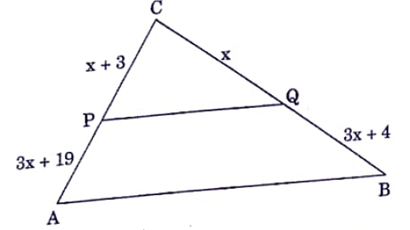1. 2
2. 3
3. 4
4. 5

Question 12.1.2.3. 0
4.Question 13.

In a single throw of a die, the probability of getting a composite number is :

1.2.3.4.Question 14.

The decimal expansion of the rational numberwill terminate after

1. One decimal place
2. two decimal places
3. three decimal places
4. four decimal places

Question 15.

The pair of lines represented by the linear equations 3x + 2y = 7 and 4x + 8y - 11 = 0 are

1. perpendicular
2. parallel
3. intersecting
4. coincident

Question 16.

In an equilateral triangle with length of side p, the length of the altitude is :

1.2.3.4.Question 17.

Given that sin θ =, tan θ is equal to :

1.2.3.4.Question 18.

A vertical pole of length 19 m casts a shadow 57 m long on the ground and at the same time a tower casts a shadow 51 m long. The a height of the tower is:

1. 171m
2. 13 m
3. 17 m
4. 117 m

Question 19.

The simplest form ofis

1. cos θ
2. sin θ
3. cot θ
4. tan θ

Question 20.

In the given figure, ∠ABC and ∠ ACB are complementary to each other and AD ⊥ BC. Then,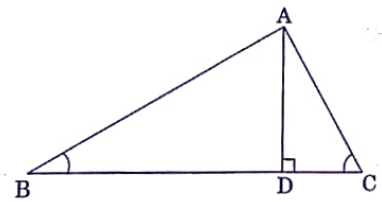1. BD.CD = BC2
2. AB.BC = BC2

SECTION B

Questions no. 21 to 40 are of 1 mark each. Answer any 16 questions from Q. No. 21 - 40.

Question 21.

If one of the zeroes of a quadratic polynomial (k - 1) x2 + kx + 1 is -3, then the value of k is:

1.2.3.4.Question 22.

If the lengths of diagonals of a rhombus are 10 cm and 24 cm, then the perimeter of the rhombus is:

1. 13 cm
2. 26 cm
3. 39 cm
4. 52 cm

Question 23.

In the given figure, x expressed in terms of a, b, c, is :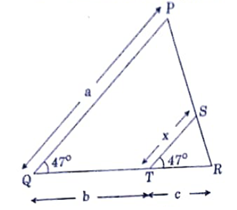1.2.3.4.Question 24.1. 0
2. 1
3. sin θ + cos θ
4. sin θ – cos θ

Question 25.

If ‘n' is any natural number, then (12)n cannot end with the digit:

1. 2
2. 4
3. 8
4. 0

Question 26.

A wire can be bent in the form of a circle of radius 56 cm. If the same wire is bent in the form of square, then the area of the square will be:

1. 8800 cm2
2. 7744 cm2
3. 6400 cm2
4. 3520 cm2

Question 27.

The probability that a non-leap year has 53 Wednesday, is :

1.2.3.4.Question 28.

If the given figure, points A, B, C and D are concyclic and ∠CBE = 130°. Then ∠FDC is :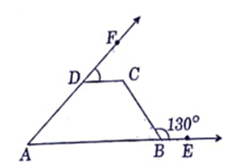1. 130°
2. 80°
3. 50°
4. 30°

Question 29.
The x – coordinate of a point P is twice its y-coordinate. If P is equidistant from Q(2, -5) and R(-3, 6), then the coordinates of P are :
1. (8, 16)
2. (10, 20)
3. (20, 10)
4. (16, 8)

Question 30.

If the point (x, 4) lies on a circle whose centre is at origin and radius is 5 cm, then the value of x is :

1. 0
2. ±4
3. ±5
4. ±3

Question 31.

The value of θ for which 2 sin 2θ = 1, is :

1. 15°
2. 30°
3. 45°
4. 60°

Question 32.

The number 385 can be expressed as the product of prime factors as

1. 5×11×13
2. 5×7×11
3. 5×7×13
4. 5×11×17

Question 33.

The difference between circumference and radius of a circle is 111 cm. The area of the circle is

1. 1366 cm2
2. 1386 cm2
3. 1376 cm2
4. 1396 cm2

Question 34.

From the letters of word ‘MANGO’, a letter is selected at random. The probability that the letter is a vowel, is :

1.2.3.4.Question 35.

If 17x – 19y = 53 and 19x – 17y = 55, then the value of (x + y) is :

1. 1
2. -1
3. 3
4. -3

Question 36.

The ratio in which the point ( -4, 6) divides the line segment joining the points A (-6, 10) and B(3, -8) is :

1. 2:5
2. 7:2
3. 2:7
4. 5:2

Question 37.

If sin2θ + sinθ = 1, then the value of cos2θ + cos4θ is :

1. -1
2. 1
3. 0
4. 2

Question 38.

38.The decimal expression of:

1. is terminating
2. is non-terminating and non-recurring
3. is non-terminating and recurring
4. does not exist

Question 39.

If the circumference of a circle is tripled, then its area becomes:

1. three times
2. nine times
3. eight times
4. two times

Question 40.

A father is three times as old as his son. In 12 years time, he will be twice as old as his son. The sum of the present ages of the father and the son is:

1. 36 years
2. 48 years
3. 60 years
4. 42 years

SECTION C
(Case Study Based Questions)
Section C consists of 10 questions of 1 mark each. Attempt any 8 questions from Q. No. 4150.                8 × 1 = 8

(Case Study – I)

A car moves on a highway. The path it traces is given below: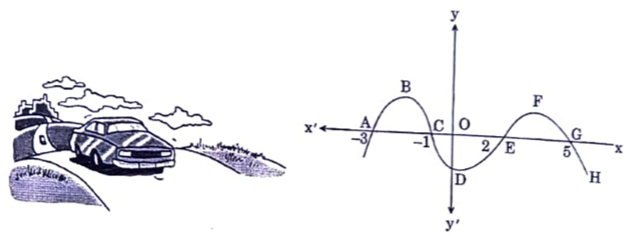Based on the above information, answer the following questions:

Question 41.

What is the shape of the curve EFG?

1. Parabola
2. Ellipse
3. Straight line
4. Circle

Question 42.

If the curve ABC is represented by the polynomial –(x2 + 4x + 3), then its zeroes are:

1. 1 and -3
2. -1 and 3
3. 1 and 3
4. -1 and -3

Question 43.

If the path traced by the car has zeroes at -1 and 2, then it is given by:

1. x2 + x + 2
2. x2 - x + 2
3. x2- x – 2
4. x2 + x – 2

Question 44.

The number of zeroes of the polynomial representing the whole curve, is:

1. 4
2. 3
3. 2
4. 1

Question 45.

The distance between C and G is:

1. 4 units
2. 6 units
3. 8 units
4. 7 units

(Case Study – II)

Shivani is an interior decorator. To design her own living room, she designed wall shelves. The graph of intersecting wall shelves is given below: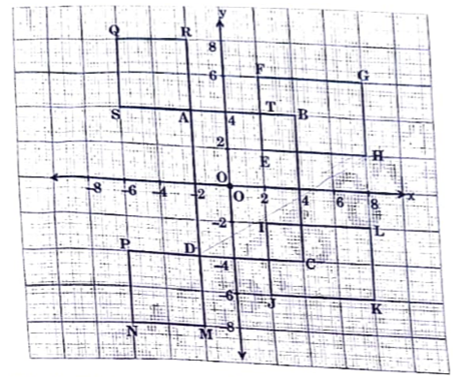Based on the above information, answer the following questions:

Question 46.

If O is the origin, then what are the coordinates of S?

1. (-6, -4)
2. (6, 4)
3. (-6, 4)
4. (6, -4)

Question 47.

The coordinates of the mid-point of the line-segment joining D and H is:

1.2. (3, -1)
3. (3, 1)
4.Question 48.

The ratio in which the x-axis divides the line-segment joining the points A and C, is:

1. 2 : 3
2. 2 : 1
3. 1 : 2
4. 1 : 1

Question 49.

The distance between the points P and G is

1. 16 units
2. 3√74 units
3. 2√74 units
4. √74 units

Question 50.

The coordinates of the vertices of rectangle IJKL are:

1. I(2, 0), J(2, 6), K(8, 6), L(8, 2)
2. I(2,-2), J(2, -6), K(8, -6), L(8, -2)
3. I(-2, 0), J(-2, 6), K(-8, 6), L(-8, 2)
4. I(-2, 0), J(-2, -6), K(-8, -6), L(-8, -2)